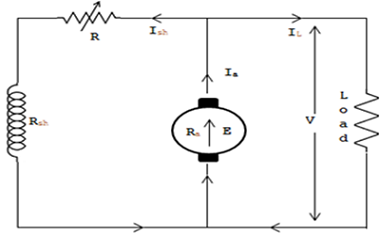# Equation for Currents and Voltages in DC Generator

Relation between Currents and Voltages:

Let, IL be the load current in amperes
Ise be the series field current in amperes
Ish be the shunt field current in amperes
Ia be the armature current in amperes
Ra be the armature resistance in ohms
Rsh be the shunt field resistance in ohms
Rse be the series field resistance in ohms
Eg be the generator e.m.f. in volts
V be the terminal voltage in volts

Then the equation for currents and voltages are given in the succeeding paragraphs.

1. Equation for D.C. Shunt Generator :

In a D.C. shunt generator the load resistance is connected across the armature brushes. So also the field coils. The current from the generator brushes is split into two components, one for the shunt field coil and the other for the load. The armature resistance is shown inside the brushes for the sake of calculation and there would be a definite voltage drop across the armature resistance.

As seen from Figure,

Ia = IL + Ish

The load voltage will be less than the generated voltage.
This is because of the voltage drop in the armature circuit.

Eg = VL + IaRaDC Shunt Generator on Load
2. Equation for D.C. Series Generator :

In a D.C. series generator, the field coils are connected in series to the armature. Hence, the field resistance (Rse) will act in series to armature resistance (Ra). The load is connected across the armature and series field. The current in the series field and load are same i.e.,
Ia = Ise = IL

But the voltage equal is as under

Eg - laRse - IaRa = V
or Eg - la (Rse + Ra) = V
or Eg - IL (Rse + Ra) = VDC Series Generator
3. D.C. Compound Generator :

A D.C. compound generator is a combination of shunt and series generators. If the shunt field is connected across the armature, it is called short shunt compound generator and when the shunt field is connected across the armature and field, i.e., across the load, it is called long shunt compound generator. The equation for compound generator is as follows :

Equation for Short shunt compound generator :

Ia = Ish + IL
and V + ILRse = Vsh
Vsh + IaRa = Eg
or Eg = V + Ise Rse + IRa